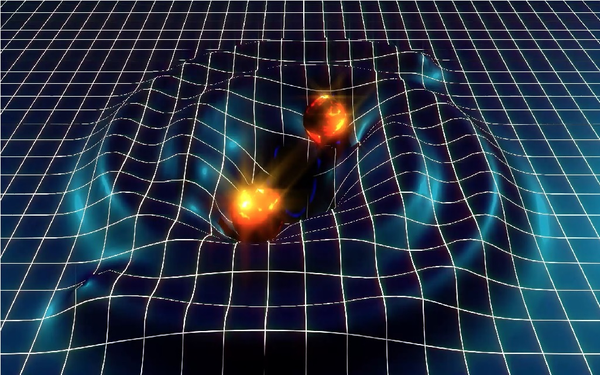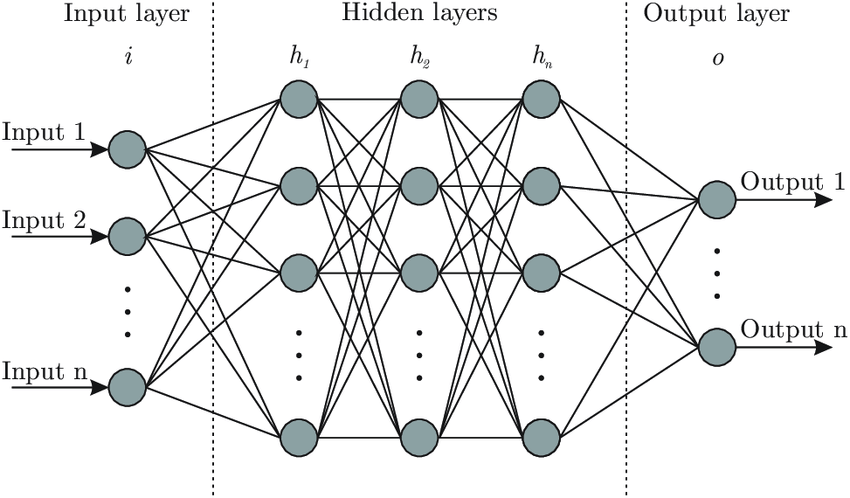en-us
• es
• en-us# The fundamental and the complex

03/24/2020

Quantum physics is the set of theories which describe the behavior of the fundamental particles that make up the physical world. However, there is a problem when we try to apply these theories to systems with a lot of particles. This is referred to as the many-body problem, and it arises due to the fact that the amount of quantum information that describes a physical system increases exponentially as we increase the number of particles which constitute it. The reason for this is that the interactions between particles create entanglement, making the wave function (a function which contains all the quantum information of a system) of the set of particles too complicated for any exact or analytical calculations to be made.

The motivation to solve this problem is none other than to gain a better understanding of how fundamental particles interact with each other to form the complex world that we see around us. It is simply fascinating to think about how the apparently simple particles of the Standard Model can give rise to an organism that can create poetry and can actually learn how those particles that he is made of behave. I deeply encourage you to take a moment to savor this wonderful picture.

In order to be fully aware of the dimensions of the many-body problem, we can use the example of a human cell. Each of our cells has approximately 100 trillion atoms, and each of these atoms is made up of more fundamental particles. Knowing that we are still unable to describe the wave function of small sets of particles, the idea of representing complex bodies like a human cell from the fundamental theories of quantum physics is simply too idealistic at the moment. The amount of quantum information that we would have to deal with is simply beyond our current computational capabilities, and so, for a long time, we weren't able to model the wave function of many-body systems.However, in the scientific paper "Solving the quantum many-body problem with artificial neural networks", published in 2017 by Giuseppe Carleo and Matthias Troyer, they argue that artificial neural networks (ANNs) can reduce the wave function down to its essential characteristics. In this way, ANNs which have previously analyzed quantum data of a many-body system are able to "learn" how to replicate the behavior of the wave function of this system. These neural networks therefore become an approximation of the wave function itself in the sense that, given some input many-body configurations (degrees of freedom of the quantum system like spins), they will return a series of exponentially many complex numbers that fully specify the amplitude and the phase of the quantum state. The relevance of this approach is that it can be used to solve some specific scenarios which cannot be represented by current numerical methods.

Until this discovery came about, the main methods used in this field have been the Quantum Monte Carlo methods (QMC) and Compression approaches, both of which are numerical techniques. Nevertheless, these methods are not capable of solving some relevant regimes such as the exact ground state (minimal energy state) properties of strongly interacting fermions (particles with half-integer spins) and the dynamical properties of high dimensional systems. And it is precisely in this article where it is shown that ANNs have a lot to say in some of these scenarios which are out of reach of numerical approaches.

ANNs are computational models which have the ability to find patterns in a set of data and to reproduce that learned behavior. The basic idea was introduced by Warren McCulloch and Walter Pitts in 1943, but it wasn't until the 2000s that significant developments were made. Nowadays, they are used in all sorts of areas like economics, speech recognition, image recognition, data analysis, etc. They are formed by computational neurons, similar to their biological namesakes in the fact that the output information of one neuron is the input of another neuron. These are in turn organized into different layers, each of which carries out a specific function. As a general structure, ANNs have an input layer (which receives the input data), a hidden layer (which weighs the inputs, sums the weighted inputs and passes the weighted sum through an activation function), and an output layer (which returns the outputs).Two of the advantages of representing the wave function with ANNs are that the accuracy of the predictions increases as we increase the number of neurons in the hidden layer, and that the representation can conserve the symmetries of the system. The mathematical foundations of why neural networks have this ability to represent extremely complicated systems are, in fact, the representability theorems.

The way in which these neural networks "learn" in this specific investigation, is by reinforcement learning. This is a type of machine learning based on maintaining a balance between the exploitation of known patterns, and the exploration of new possibilities for a better long-term knowledge of the system. The ANN optimizes its weighting parameters after each iteration to minimize the error of the prediction. This reduction of the quantum information allows Schrödinger's equation for a many-body system to be solved with a low computational cost and with a high level of accuracy for both ground-state (minimal energy state) properties and out of equilibrium dynamics. This is shown with the representation of two prototypical spin models (Ising and Heisenberg) in 1D and 2D. In 1D, the accuracy of the ANN approach is the same as the best current methods (like MPS representation), but with way fewer parameters, while in 2D, on the other hand, the accuracy is the best reported to date.

Some further implications of this investigation are that, due to a better understanding of the essential characteristics of the wave function, new concepts related to quantum information theory could be discovered; and that, even though this specific paper is focused on interacting spins systems, it could be extended to tackle other quantum systems. Also, the fact that they used a restricted Boltzman machine architecture (a simple ANN) raises the question of what more advanced and modern ANNs - like deep network architectures and convolutional neural networks - are capable of.

To summarize, the main achievement of this scientific paper is that it introduces a representation of the wave function in terms of artificial neural networks. This makes it possible to solve the many-body problem for some relevant scenarios which couldn't be solved before. The parameters of the neural network are optimized using reinforcement learning to best represent the wave function; an approach which they have validated by studying the Ising and Heisenberg models in both one and two dimensions. Because the accuracy of the predictions can be improved by simply adding more neurons to the ANN, the power of artificial neural networks in this field has been demonstrated.

We live in a world that is governed by the laws of the very small, but this is something which I find incredibly hard to grasp. Electrons, protons, photons... they are all the ultimate reason for the colors of the sunset, the birth of a child and the behavior of a jaguar. Let the beauty of these incredibly complex systems lead us to realize the beauty of the fundamental building blocks of nature, and of nature as a whole.

*BIBLIOGRAPHY

• Carleo, Giuseppe; Troyer, Matthias. "Solving the quantum many-body problem with artificial neural networks", Science, Vol. 355 (February 2017).
• H. Hassoun, Mohamad. Fundamentals of Artificial Neural Networks (The MIT Press, 2003).

JOSÉ GEFAELL BERENGUER